### 4. EXTREME VALUE THEORY AND BRIGHTEST GALAXIES

This is an application of the statistical theory of extreme values to astronomy. Once again the name of the illustrious statistician Sir Ronald Fisher comes up. for it was he who developed the theory of extreme values. The problem has to do with the remarkably small dispersion (0.35 mag) in the magnitudes, M1, of the first brightest galaxies in clusters. This has made them indispensable as "standard candles", and they have, for this reason, become the most powerful yardsticks in observational cosmology. There has existed, however disagreement as to the nature of these objects. The two opposing viewpoints have been: that they are a class of "special" objects (Peach 1969; Sandage 1976; Tremaine and Richstone 1977); and at the other extreme, that they are "statistical", representing the tail end of the luminosity function for galaxies (Peebles 1968, Geller and Peebles 1976).

In 1928, in a classic paper, R.A. Fisher and L.H.C. Tippett had derived the general asymptotic form that a distribution of extreme sample values should take - independent of the parent distribution from which they are drawn! This work was later expanded upon by Gumbel (1958).

From extreme value theory, for clusters of similar size, the probability density distribution of M1 for the "statistical" galaxies is given (Bhavsar and Barrow 1985) by the distribution in equation 6, which is often referred to as the first Fisher-Tippett asymptote, or Gumbel distribution.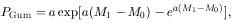(6)

where a is the parameter which measures the steepness of the luminosity function at the tail end. M0 is the mode of the distribution and is a measure of the cluster mass, but with only a logarithmical dependence on the cluster mass.

We can compare the above distribution with the data to answer the question: "Are the first-ranked galaxies statistical?" Figure 9 shows the maximum likelihood fit of equation (6) to the magnitudes of the 93 first-ranked galaxies from a homogeneous sample of richness 0 and 1 Abell clusters. This excellent data for M1 was obtained by Hoessel, Gunn and Thuan (1980, [HGT]). The fit is very bad. A Kolmogorov-Smirnov test also rejects the statistical hypothesis with 99% confidence. If all the galaxies are special, it is not possible to have a theoretical expression for their distribution in magnitude. Though we can make a simple argument that if they are formed from normal galaxies as a standard "mold", we expect a Gaussian distribution for their magnitudes or luminosities. This is in fact what is assumed for their distribution by most observers, as seen from the available literature. This possibility is explored also, and figure 9 shows a Gaussian with the same mean and variance as the data compared with the data for both cases where the magnitudes or the luminosities have a Gaussian distribution. The case where the luminosities are distributed as a Gaussian is called expnormal (in analogy to lognormal) for the magnitudes. Neither, it can be seen from figure 9 is acceptable.

It was shown that a "two population model" (Bhavsar 1989) in which the first brightest galaxies are drawn from two distinct populations of objects - a class of special galaxies and a class of extremes of a statistical distribution - is needed. Such a model can explain the details of the distribution of M1 very well. Parameters determined purely on the basis of a statistical fit of this model with the observed distribution of the magnitudes of the brightest galaxies, are exceptionally consistent with their physically determined and observed values from other sources.

The probability density distribution of the magnitudes of the special galaxies is assumed to be a Gaussian. This is the most general expression for the distribution of either the magnitudes or luminosities of these galaxies, if they arise from some process which creates a standard mold with a small scatter, arising because of intrinsic variations as well as experimental uncertainty in measurement. The distribution of M1 for the special galaxies is given by(7)

If a fraction, d, of the rich clusters have a special galaxy which competes with the normal brightest galaxy for first-rank, then the distribution that results is derived in Bhavsar (1989). This expression, f (M1), which describes the distribution of M1 for the first-ranked galaxies in rich clusters, for the two population model is given by equation (8)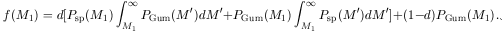(8)

The first term in the above expression gives the probability density distribution of special galaxies with the condition that the brightest normal galaxy in that cluster is always fainter. The second term gives the probability density distribution of first-ranked normal galaxies, in clusters containing a special galaxy, but the special galaxy is always fainter. The last term gives the probability density of normal galaxies in clusters that do not have a special galaxy. Equation (8) is our model's predicted distribution of M1 for the brightest galaxies in rich clusters. The parameters in this model are: i)sp - the standard deviation in the magnitude distribution of the special galaxies; ii) Msp - the mean of the absolute magnitude of the special galaxies; iii) a - the measure of the steepness of the luminosity function of galaxies at the tail end; iv) Mex - the mean of the absolute magnitude of the statistical extremes given by Mex = M0 - .577/a, we shall instead use the parameter b = Msp - Mex, the difference in the means of the magnitudes of special galaxies and statistical extremes; and v) d - the fraction of clusters that have a special galaxy.

We have chosen the maximum-likelihood method, being the most bias free, and therefore best suited, to determine the values of the parameters. There are five independent parameters and 93 values of data. We maximize the likelihood function, defined by(9)

where the function f (M1[i]) is the value of f (M1) defined in equation (8), evaluated at each of the 93 values of M1 respectively for i = 1 to 93. The values of the parameters that maximize £ give the maximum-likelihood fit of the model to the data. The parameters, thus determined, have the following values:(10)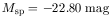(11)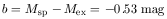(12)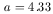(13)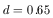(14)

Figure 10 compares the data to the model [equation (8)] evaluated for the parameter values determined above. The fit is very good. Note that the fit is calculated using all 93 independent observations, and not tailored to fit this particular histogram.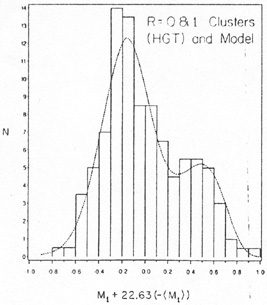Figure 10.

A further detailed statistical analysis by Bhavsar and Cohen (1989) of alternatives to the assumed Gaussian distribution of the magnitudes of special galaxies, along with a study of the confidence limits of the parameters determined by maximum-likelihood and other statistical methods has determined a "best" model. It turns out that the models in which the luminosities have a Gaussian distribution work marginally better. This may have been expected, luminosity being the physical quantity. This model requires 73% of the richness 0 and 1 clusters to have a special galaxy which is on average half a magnitude brighter than the average brightest normal galaxy. As a result, about 66% of all first-ranked galaxies in richness 0 and 1 clusters are special. This is because in 7% of the clusters, though a special galaxy is present, it is not the brightest.

Although it is generally appreciated that some of the brightest galaxies in rich dusters are a morphologically distinct class of objects (eg cD galaxies); we have approached the problem from the viewpoint of the statistics of their distribution in M1, and conclude that indeed some of the brightest galaxies in rich clusters are a special class of objects, distinct from the brightest normal galaxies. Further we have been able to model the distribution of these galaxies. We have presented statistical evidence that the magnitudes of first-ranked galaxies in rich clusters are best explained if they consist of two distinct populations of objects; a population of special galaxies having a Gaussian distribution of magnitudes with a small dispersion (0.21 mag), and a population of extremes of a statistical luminosity function. The best fit model requires that 73% of the clusters have a special galaxy that is on average 0.5 magnitudes brighter than the brightest normal galaxy. The model also requires the luminosity function of galaxies in clusters to be much steeper at the very tail end, than conventionally described.